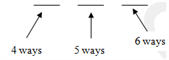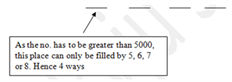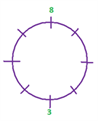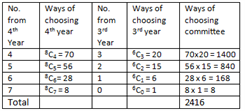# Permutation and Combination Solutions

Detailed solution of Permutaion and Combination

1)12x[n!/(n – 6)!]=n!/(n – 4)!

➜ 12(n – 4)! = (n – 6)! ➜ 12 (n – 4)! = (n – 6) (n – 5) (n – 4)!

➜ 12 = n2 – 11n + 30 ➜ n2 – 11n + 18 = 0

➜ (n – 9) (n – 2) = 0 n = 9, n = 2

Neglecting n = 2, n = 9.

Hence option (c)

2) We have nCr-1 + nCr = n + 1Cr

7Cx-1 + 7Cx = 8Cx➜8Cx = 8Cx+2

➜ Either x = x + 2 or x + x + 2 = 8But x ≠ x+2

➜ 2x + 2 = 8 ➜ x = 3

Hence option (b) Alternatively, go from answer options

3) Maximum value of 16Cx occurs when x = 16/2 = 8.

Hence option (d)

4) (nPr)/(nCr )=120/5 = 24➜nCr = nPr/r!

➜nPr/[n<subPr/r!] = 24➜r! =24

➜ r = 4

Given nPr = 120

➜nP4 = 120 ➜n!/(n-4)!= 120

➜n(n-1)(n-2)(n-3) = 120 ➜ n = 5

Hence option (d)

5) This is a type of “Different to Different” question. 5 prizes can be distributed among 4 students in 45 ways.

Hence option (a)

6) Suppose we start with the units place. Unit place can be filled by any of the 6 digits given, so it can be filled in 6 ways. Now coming the tens place,

it can be filled by any of the six digits except the one which has been used at units place. So, it can be filled in 5 ways.

Similarly the hundred’s place can be filled by any of the six digits except those two which have been used at unit’s and tens’ place.

So, it can be filled in 4 waysSo, total numbers of numbers possible = 6 x5x4 = 120. Hence option (a)

Hence option (a)

Solutions for questions 7-87) When none of the digits are repeated:

The hundreds place can be filled by any of the digits: 2, 3, 5, 6, 7 or 8 except the one which has already been used at the thousands place,

so it can be filled in 5 ways. Similarly tens place can be filled in 4 ways:

only those 4 numbers which have not been use either at hundred’s or thousand’s place.

Units place can be filled in only 3 ways.

So, total number of nos. Possible = 4x 5x4x3 = 240

Hence option (b)

8) When the repetition is allowed:

All the places: Hundreds, ten’s and units can be filled by any of the digits: 2, 3, 5, 6, 7 or 8 i.e. in 6 ways. Thousand’s place can be filled only in 4 ways.

Hence no. numbers possible = 4x6x6x6 = 864.

Hence option (d)

9) We need to arrange 2 Cs, O, 3Ns, V, 3Es and I. This is permutation with repetition. It can be done in 11!/[(2!)(3!)(3!)] ways.

Hence option (c)

10) NN_ _ _ _ _ _ _EE The 7 places have to be filled by 2 Cs, O, N, V, E and I. This can be done in 7!/2!

Hence option (d)

11) Total points including the vertices = 13 Out of these 13 points, 5 points on side AB ( including B and A) are collinear 7 points on side CA (including C and A) are collinear 4 points on side BC (including B and C) are collinear Thus, total triangles possible 13C3 – 5C3 – 7C3 – 4C3 = 237 Out of these,

we should exclude those triangles which do not share theVertices A or B or C Such triangles = 13 – 3C3 – 5 – 2C3 – 7 – 2C3– 0 = 109

We should also exclude the triangle ABC itself. Hence, the answer is 237 – 110 = 127.

Hence option (d)

12) First we fix A as the first letter No. of words possible with A as the first letter = 4! = 24

No. of words possible with M as the first letter= 4!/2! = 12

No. of words possible with RAA as the first 3 letters = 2

No. of words possible with RAM as the first 3 letters = 2

As of now, we have reached 24+12+2+2= 40

Next word will be RASAM

Rank= 41

Hence option (c)

13) 108<n<109 n is a 9 digit number Product of 6 is possible in the following cases

1) Exactly one digit is 6 and the remaining digits are 1 Number of possible numbers= 9

2) One digit is 2 and the other is 3, remaining are 1 = 9P2 = 72

Total possible numbers =72+9 = 81

Hence option (c)

14) Answer option c Lets fix number 8 at a slot at the table, automatically, number 3 also gets fixed.

Out of the remaining 6 delegates, 4 and 5 will be together and can be arranged in 2! ways amongst each other.

Also for each of these ways, number 1 can sit next to 4 in only one way. Now 1,4,5 can be placed only in 2 ways(See below).This question now becomes the arrangement of the remaining 3 people x 4= 3! x 4.

Hence option (c)

15) There are a total of 8 balls with 3 of one kind, 2 of other kind and rest 3 of the same kind.

These can be arranged in 8!/((3!)(2!)(3!)) = 560.

Hence option (c)

15) There are a total of 8 balls with 3 of one kind, 2 of other kind and rest 3 of the same kind.

These can be arranged in 8!/((3!)(2!)(3!)) = 560.

Hence option (c)

16) At least 1 orange can be selected in 25 – 1 = 31 ways.At least 1 apple can be selected in 24 – 1 = 15 ways.

Total no. of selections possible = 31 x 15 = 465.

Hence option (d)

17) Majority of fourth year means out of 7, minimum 4 should be from fourth year. Considering different possibilities:Hence option (d)

Solutions for questions 18-19

18) When no two girls are together, follow the following two steps

(i) Fix the positions of the 5 boys, with one space in between them.

This can be shown as _B1 _ B2 _ B3 _ B4 _ B5_ Thus the arrangement can be done in 5! Ways

(ii) As you can see above, 6 places (gaps) are created when the boys are fixed.

The number of ways of selection of places for the 4 girls is 6C4, and the number of ways of arrangement is 4!.

=43200

Hence option (d)

19) When all girls are together, follow the following three steps

(i) Take all the girls together as one unit. So totally there are 1+5 units (5 from the boys)

(ii) Arrange these 6 units. This can be done in 6! Ways.

(iii) Now arrange the girls among themselves in 4! Ways. Answer is option (b)

Total number of arrangements=6!x4!

Hence option (b)

20) 2 Slots for 4 from the available slots can be selected in 7C2 ways Remaining 5 slots can be filled with any of 5 and 6 in 2.2.2.2.2 = 25 ways

Total ways = 7C2 x 25 = 672

Hence option (b)

21) There are 4 rows and 5 columns. Using the one-zero method, number of paths will be 9C4 = 126 ways

Hence option (c)

22) The points should be distributed on the three lines as 6,5,5 .

6C2(5C1+5C1) + 5C2(6+5) + 5C2(6+5)=520

Alternatively, 16C3-5C3-5C3-6C3=520

Hence option (c)

23) From the digits 0 1 2 3 4 5 6 7 8 9

The sum is 45

Thus, every time we remove 2 digits which add up to 9, we will have a new set of 8 numbers which are divisible by 9

Case 1

Removing 0 and 9 Number of cases possible = 8!

Case 2

Removing

(1) 8 and 1

(2) 7 and 2

(3) 6 and 3

(4) 5 and 4

In each case, total number of cases = 8!-7! (we subtract 7! Because those are the cases where 0 is the first digit) Hence,

total number of ways = 4(8!-7!)+8!= 36×7!.

Hence option (d)

24) To be divisible by 4, the last 2 digits should be divisible by 4 The combination of the last two possible digits are 12 16 24 32 36 64 for each case,

the other 2 digits can be selected and arranged in 3P2 ways = 3!=6 ways

total possible cases= 6 x 6 =36

Hence option (a)

25) Number of ways of selecting 2 couples out of 7= 7C2 Derangement of 5 couples can be done in 44 ways.

Total number of ways= 924

Hence option (b)